## PG Calculator is a powerful scientific calculator for iPhone/iPad/iPod Touch with customizable user interface.

PG Calculator works in Algebraic and RPN modes.

### Screenshots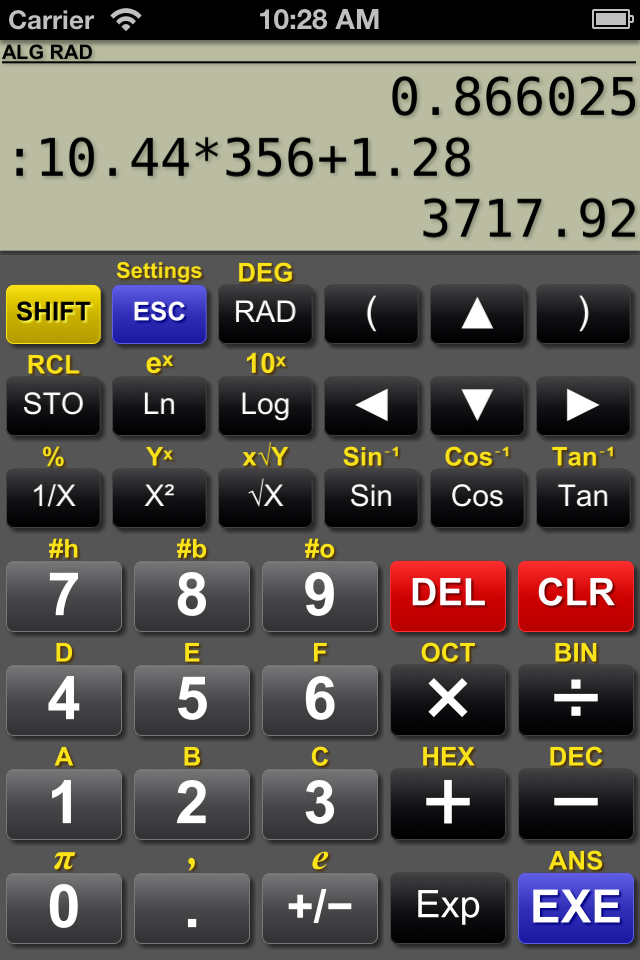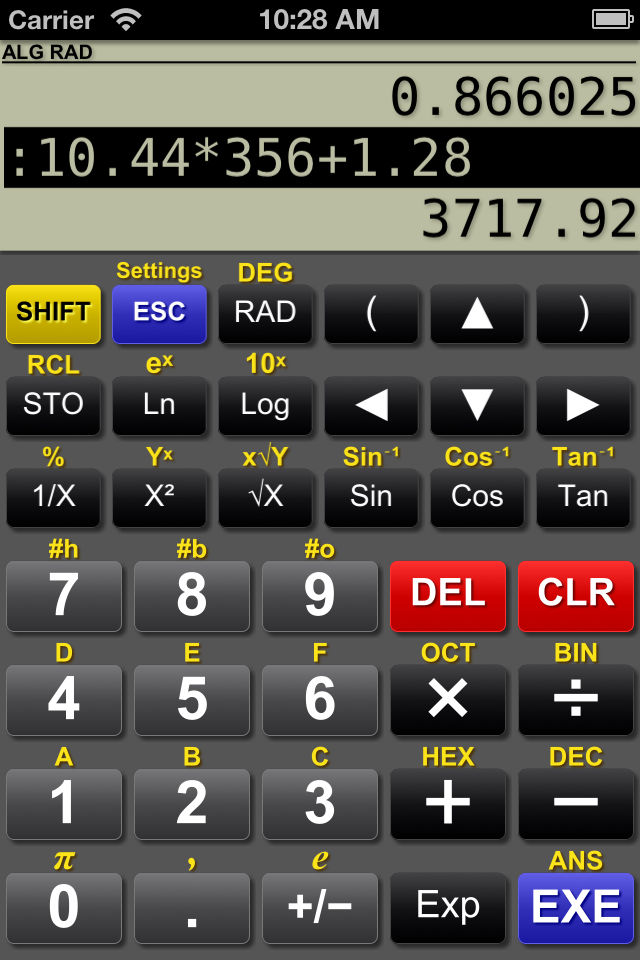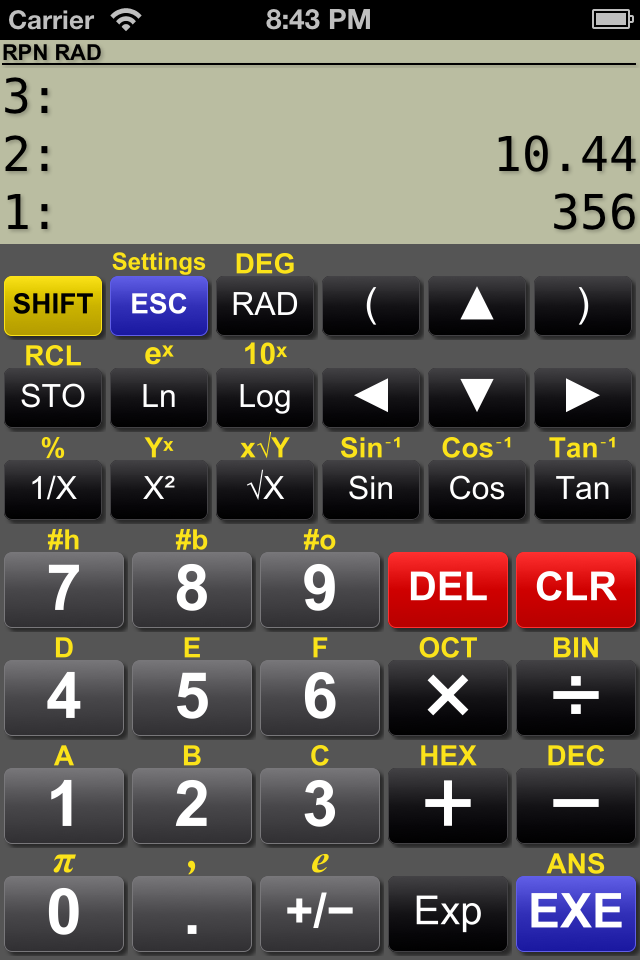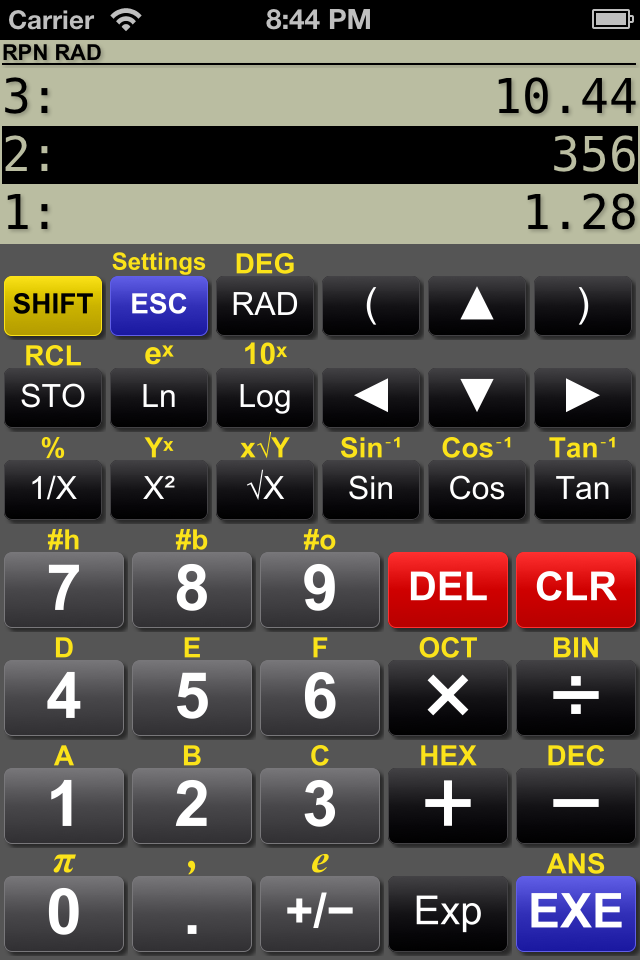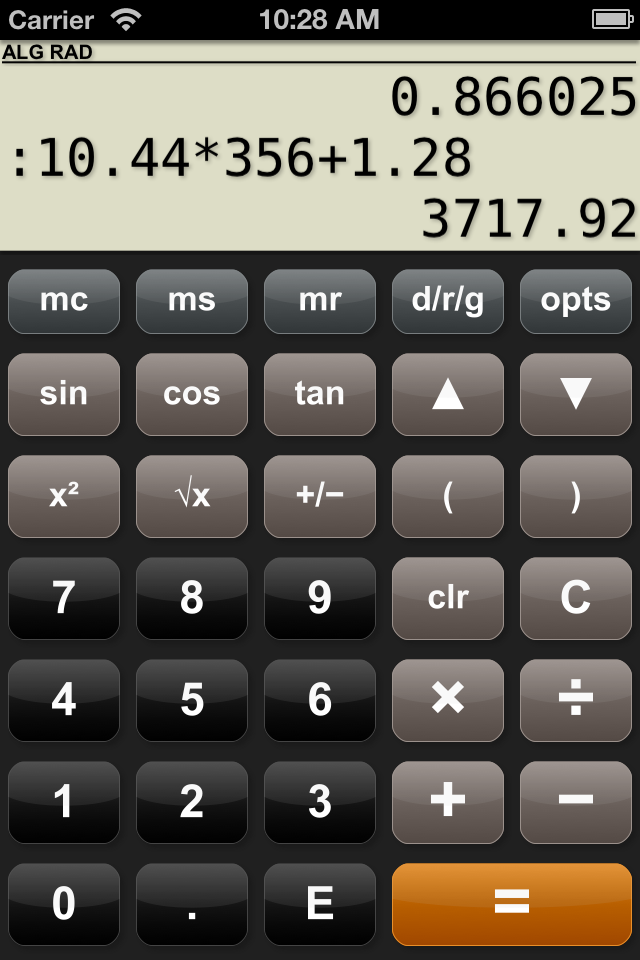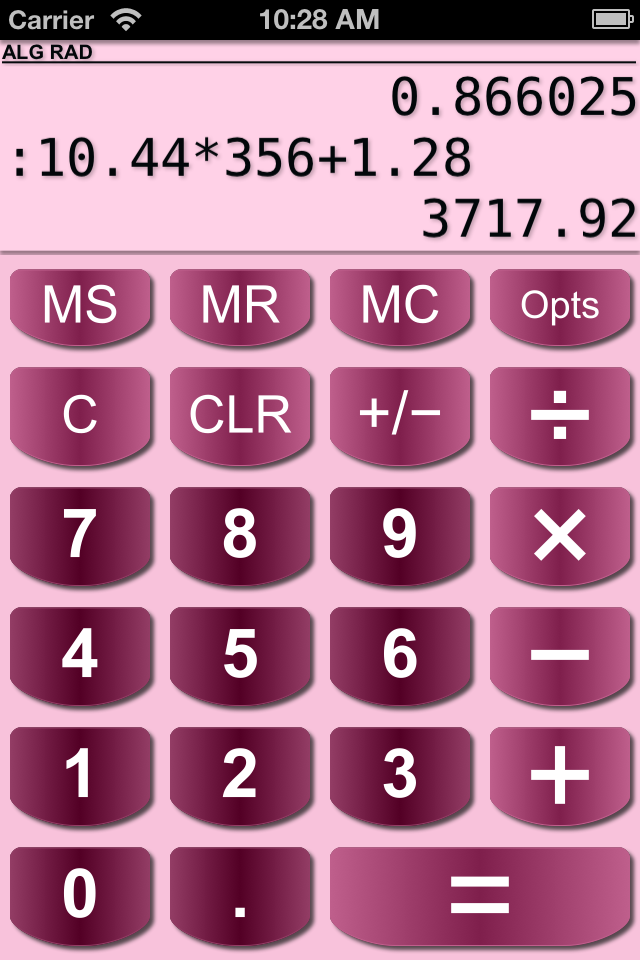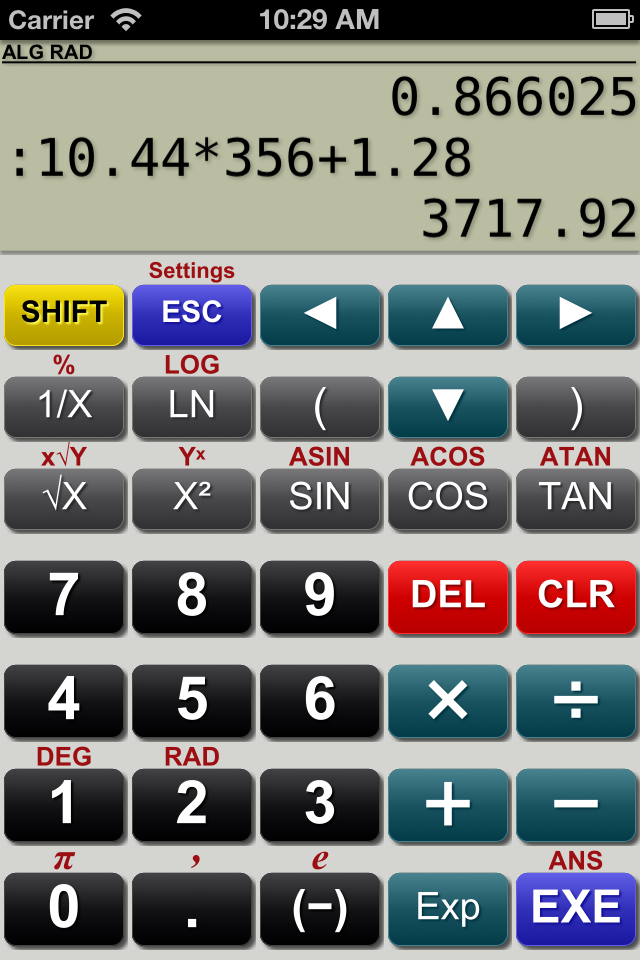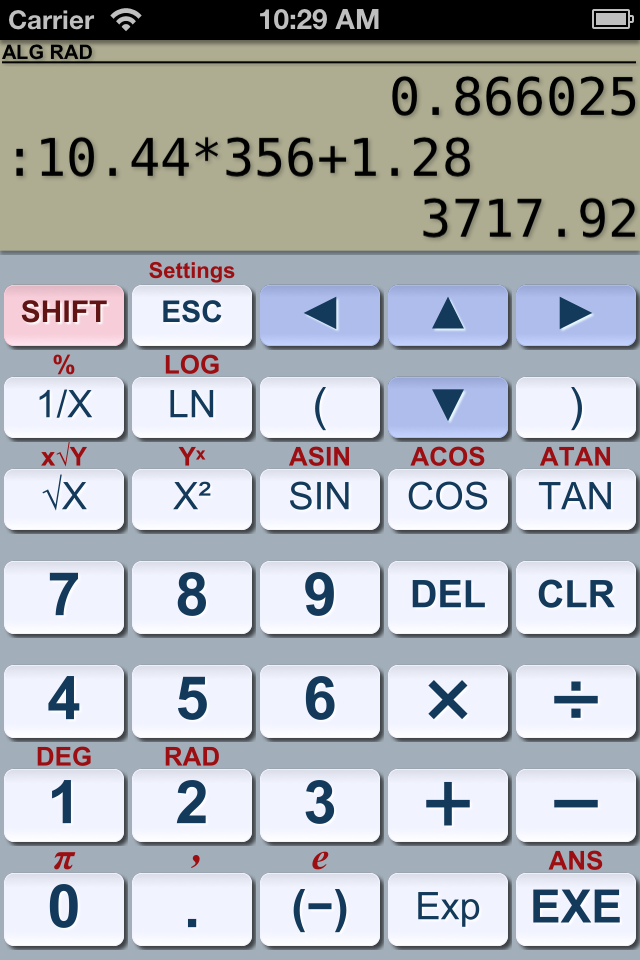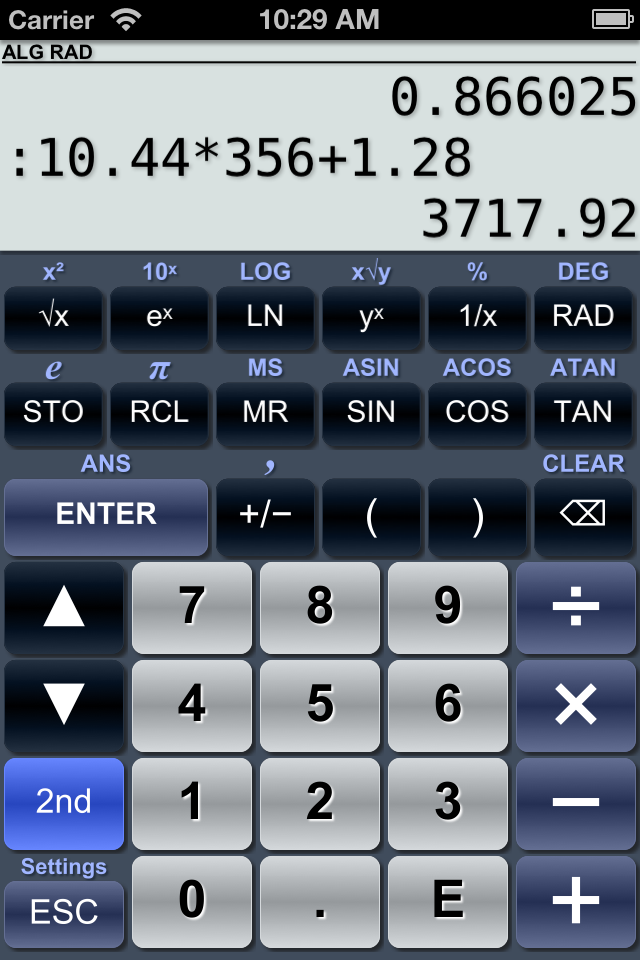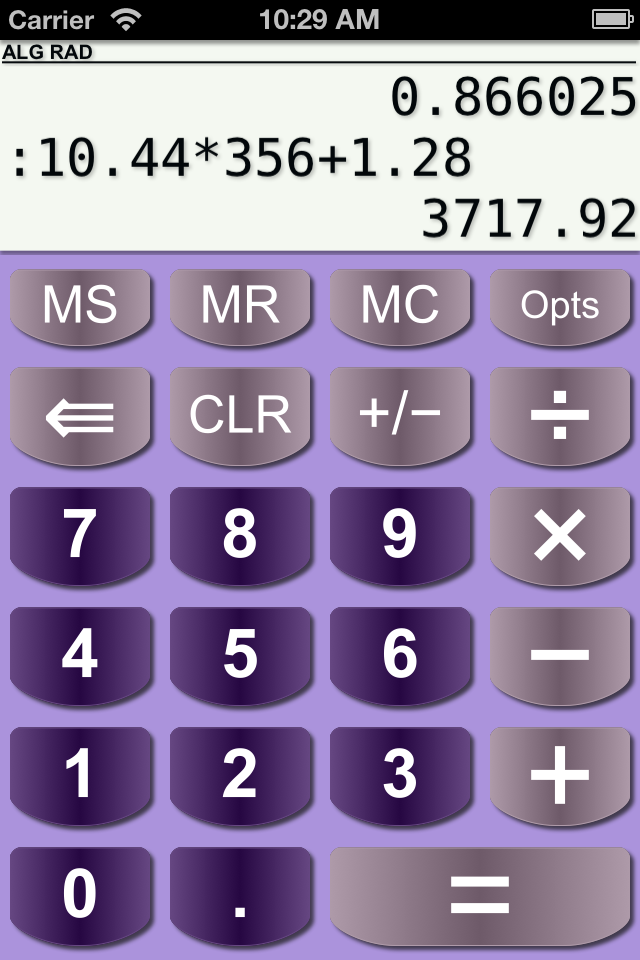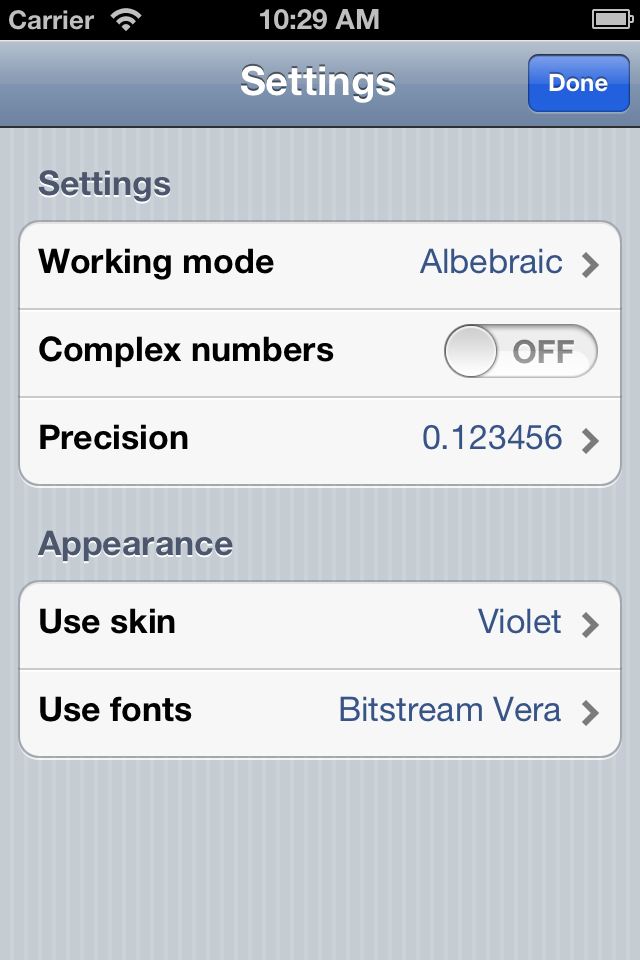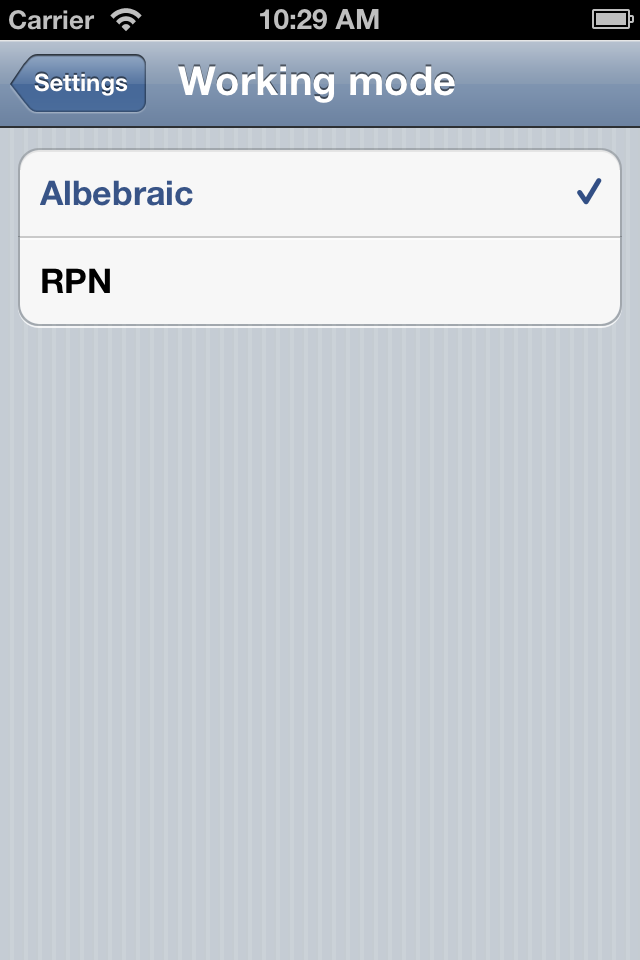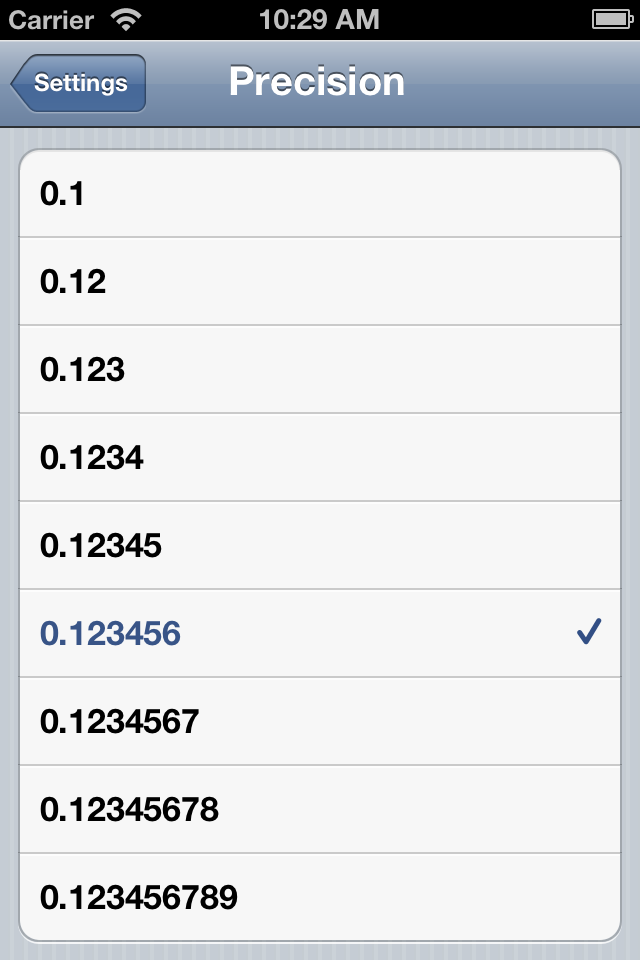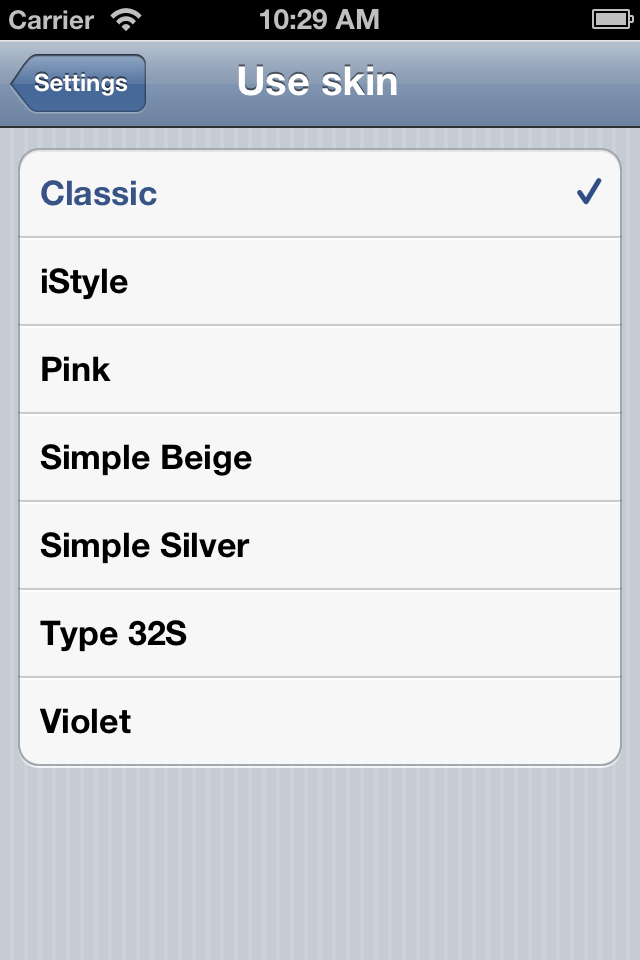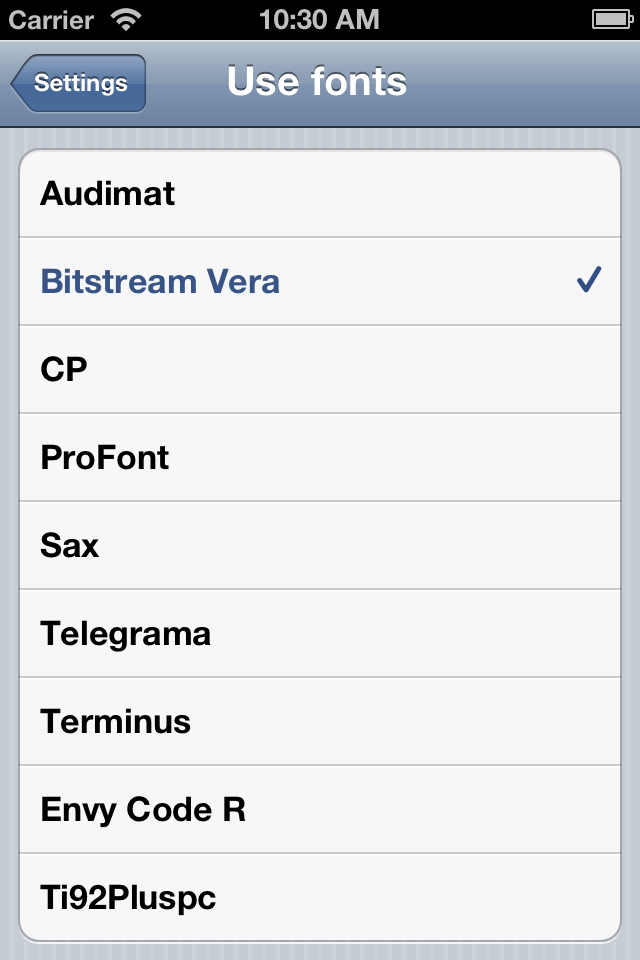PG Calculator allows to use mathematical functions described below:

• Addition, subtraction, multiplication and division;
• Power and roots;
• Trigonometric functions: sin, cos, tan, asin, acos, atan;
• Hyperbolic functions;
• natural and decimal logarithms;
• factorials;
• conversion between binary, octal, hexadecimal, decimal and scientific (exponential) number formats;

The user interface of the calculator can be changed by using various skins supplied with the application.

PG Calculator for iPhone/iPad/iPod Touch is available for following platforms:

• iOS 5 or higher;

Start a barcode scanner on your phone and scan one of the QR codes below. This will take you directly to App Store.

## PG Calculator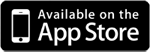You may also consider looking at PG Calculator for Windows and Linux or PG Calculator for Android